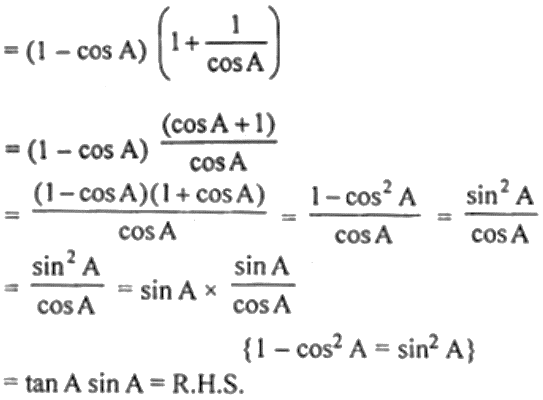Guru

# (i) tan A + cot A = sec A cosec A (ii) (1 – cos A) (1 + sec A) = tan A sin A

• 0

This ques has been taken from

Book- ML Aggarwal
Board- ICSE
Publication- Avichal
Chapter- Trigonometric Identities
Chapter number-18

We hage have to prove

(i) tan A + cot A = sec A cosec A

(ii) (1 – cos A) (1 + sec A) = tan A sin A

Ques no. 13, chapter 18th trigonometry identities

Share

1. Solution:

(i) L.H.S. = tan A + cot A

= sin A/cos A + cos A/sin A

= (sin2 A + cos2 A)/ (sin A cos A)

= 1/ (sin A cos A)

= sec A cosec A

= R.H.S

(ii) L.H.S. = (1 – cos A) (1 + sec A)• 0